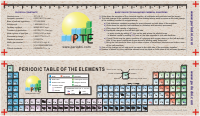# Periodic Table and other Calculators

Periodic Table and other Calculators

Periodic table of the elements contains the basic data about the elements in five languages.EniG. Chemistry Assistant 2.4 An expression calculator for fast calculation of molecular weight of compounds by simple entry of chemical formula (the element symbols can be written in lowercase letters). The calculator also provides a list of common physical constants and performs various conversions between English and metric units.

Scientific calculator Sci.Calc is a fully-functioning JavaScript calculator that contain of practically any common and many advanced calculator functions (cosine, sine, tangent, power, square root, exponents, etc.).

Learning Electronics by Hobby DIY is not Complete without a sound Knowledge of Materials, A Periodic table Reference will help in Understanding the Metals and Semiconductors.

Every Metal has a potential for a New Component Discovery, Even a Breakthrough in SuperConductivity at Room Temperature will change the way we build Gadgets !Elektrick the Power Jedi